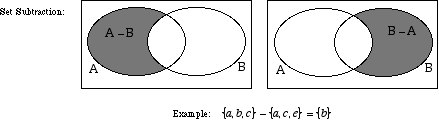index: click on a letter A B C D E F G H I J K L M N O P Q R S T U V W X Y Z A to Z index index: subject areas numbers & symbols sets, logic, proofs geometry algebra trigonometry advanced algebra & pre-calculus calculus advanced topics probability & statistics real world applications multimedia entrieswww.mathwords.com about mathwords website feedback

 Set Subtraction A way of modifying a set by removing the elements belonging to another set. Subtraction of sets is indicated by either of the symbols – or \. For example, A minus B can be written either A – B or A \ B.See also